# bayesopt

Select optimal machine learning hyperparameters using Bayesian optimization

## Syntax

``results = bayesopt(fun,vars)``
``results = bayesopt(fun,vars,Name,Value)``

## Description

example

````results = bayesopt(fun,vars)` attempts to find values of `vars` that minimize `fun(vars)`. NoteTo include extra parameters in an objective function, see Parameterizing Functions. ```

example

````results = bayesopt(fun,vars,Name,Value)` modifies the optimization process according to the `Name,Value` arguments.```

## Examples

collapse all

This example shows how to create a `BayesianOptimization` object by using `bayesopt` to minimize cross-validation loss.

Optimize hyperparameters of a KNN classifier for the `ionosphere` data, that is, find KNN hyperparameters that minimize the cross-validation loss. Have `bayesopt` minimize over the following hyperparameters:

• Nearest-neighborhood sizes from 1 to 30

• Distance functions `'chebychev'`, `'euclidean'`, and `'minkowski'`.

For reproducibility, set the random seed, set the partition, and set the `AcquisitionFunctionName` option to `'expected-improvement-plus'`. To suppress iterative display, set `'Verbose'` to `0`. Pass the partition `c` and fitting data `X` and `Y` to the objective function `fun` by creating `fun` as an anonymous function that incorporates this data. See Parameterizing Functions.

```load ionosphere rng default num = optimizableVariable('n',[1,30],'Type','integer'); dst = optimizableVariable('dst',{'chebychev','euclidean','minkowski'},'Type','categorical'); c = cvpartition(351,'Kfold',5); fun = @(x)kfoldLoss(fitcknn(X,Y,'CVPartition',c,'NumNeighbors',x.n,... 'Distance',char(x.dst),'NSMethod','exhaustive')); results = bayesopt(fun,[num,dst],'Verbose',0,... 'AcquisitionFunctionName','expected-improvement-plus')```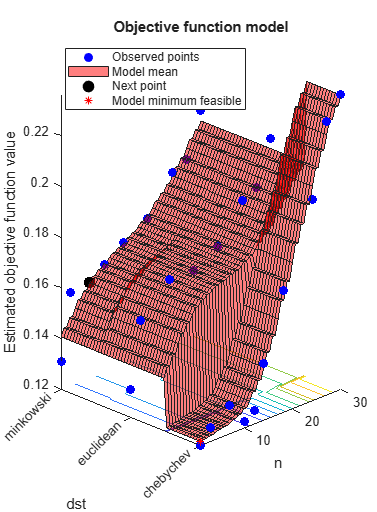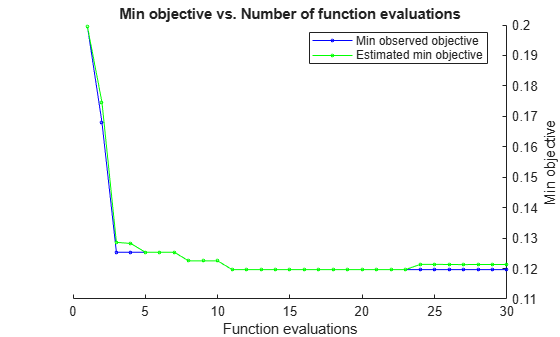```results = BayesianOptimization with properties: ObjectiveFcn: @(x)kfoldLoss(fitcknn(X,Y,'CVPartition',c,'NumNeighbors',x.n,'Distance',char(x.dst),'NSMethod','exhaustive')) VariableDescriptions: [1x2 optimizableVariable] Options: [1x1 struct] MinObjective: 0.1197 XAtMinObjective: [1x2 table] MinEstimatedObjective: 0.1213 XAtMinEstimatedObjective: [1x2 table] NumObjectiveEvaluations: 30 TotalElapsedTime: 56.0618 NextPoint: [1x2 table] XTrace: [30x2 table] ObjectiveTrace: [30x1 double] ConstraintsTrace: [] UserDataTrace: {30x1 cell} ObjectiveEvaluationTimeTrace: [30x1 double] IterationTimeTrace: [30x1 double] ErrorTrace: [30x1 double] FeasibilityTrace: [30x1 logical] FeasibilityProbabilityTrace: [30x1 double] IndexOfMinimumTrace: [30x1 double] ObjectiveMinimumTrace: [30x1 double] EstimatedObjectiveMinimumTrace: [30x1 double] ```

A coupled constraint is one that can be evaluated only by evaluating the objective function. In this case, the objective function is the cross-validated loss of an SVM model. The coupled constraint is that the number of support vectors is no more than 100. The model details are in Optimize Cross-Validated Classifier Using bayesopt.

Create the data for classification.

```rng default grnpop = mvnrnd([1,0],eye(2),10); redpop = mvnrnd([0,1],eye(2),10); redpts = zeros(100,2); grnpts = redpts; for i = 1:100 grnpts(i,:) = mvnrnd(grnpop(randi(10),:),eye(2)*0.02); redpts(i,:) = mvnrnd(redpop(randi(10),:),eye(2)*0.02); end cdata = [grnpts;redpts]; grp = ones(200,1); grp(101:200) = -1; c = cvpartition(200,'KFold',10); sigma = optimizableVariable('sigma',[1e-5,1e5],'Transform','log'); box = optimizableVariable('box',[1e-5,1e5],'Transform','log'); ```

The objective function is the cross-validation loss of the SVM model for partition `c`. The coupled constraint is the number of support vectors minus 100.5. This ensures that 100 support vectors give a negative constraint value, but 101 support vectors give a positive value. The model has 200 data points, so the coupled constraint values range from -99.5 (there is always at least one support vector) to 99.5. Positive values mean the constraint is not satisfied.

```function [objective,constraint] = mysvmfun(x,cdata,grp,c) SVMModel = fitcsvm(cdata,grp,'KernelFunction','rbf',... 'BoxConstraint',x.box,... 'KernelScale',x.sigma); cvModel = crossval(SVMModel,'CVPartition',c); objective = kfoldLoss(cvModel); constraint = sum(SVMModel.IsSupportVector)-100.5; ```

Pass the partition `c` and fitting data `cdata` and `grp` to the objective function `fun` by creating `fun` as an anonymous function that incorporates this data. See Parameterizing Functions.

```fun = @(x)mysvmfun(x,cdata,grp,c); ```

Set the `NumCoupledConstraints` to `1` so the optimizer knows that there is a coupled constraint. Set options to plot the constraint model.

```results = bayesopt(fun,[sigma,box],'IsObjectiveDeterministic',true,... 'NumCoupledConstraints',1,'PlotFcn',... {@plotMinObjective,@plotConstraintModels},... 'AcquisitionFunctionName','expected-improvement-plus','Verbose',0); ```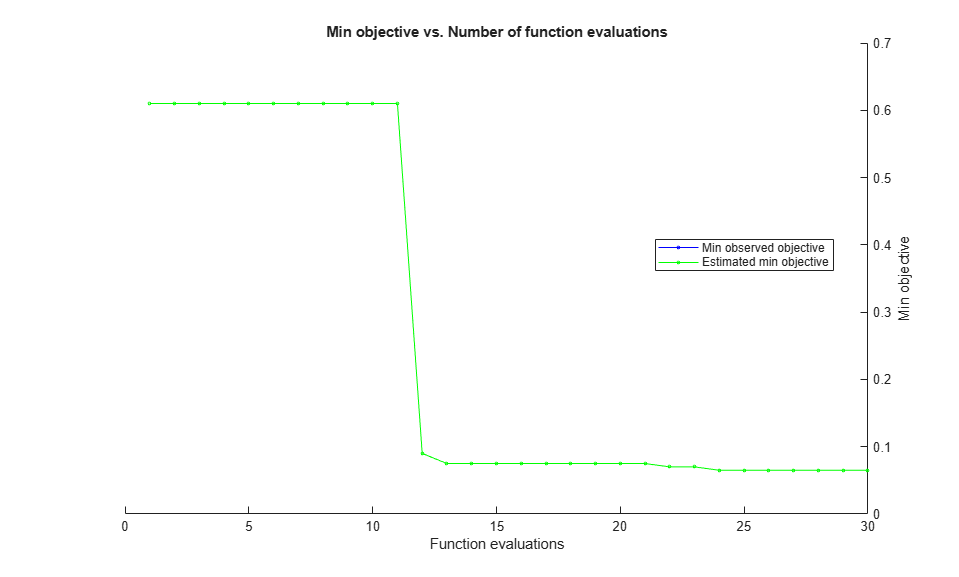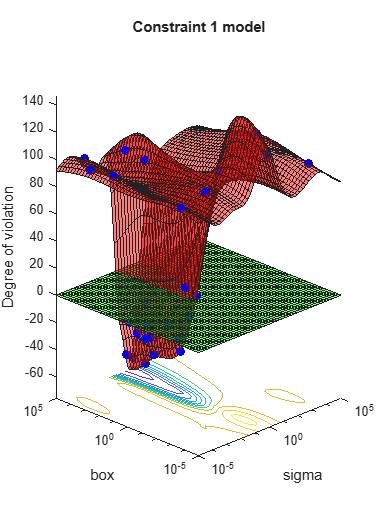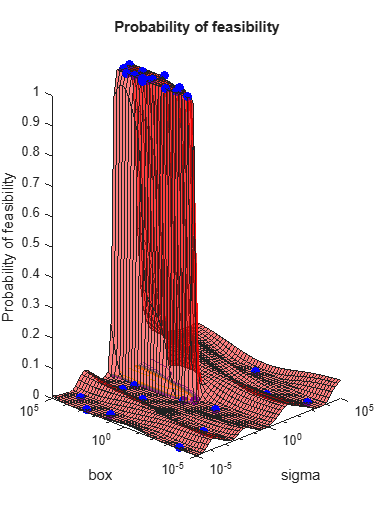Most points lead to an infeasible number of support vectors.

Improve the speed of a Bayesian optimization by using parallel objective function evaluation.

Prepare variables and the objective function for Bayesian optimization.

The objective function is the cross-validation error rate for the ionosphere data, a binary classification problem. Use `fitcsvm` as the classifier, with `BoxConstraint` and `KernelScale` as the parameters to optimize.

```load ionosphere box = optimizableVariable('box',[1e-4,1e3],'Transform','log'); kern = optimizableVariable('kern',[1e-4,1e3],'Transform','log'); vars = [box,kern]; fun = @(vars)kfoldLoss(fitcsvm(X,Y,'BoxConstraint',vars.box,'KernelScale',vars.kern,... 'Kfold',5));```

Search for the parameters that give the lowest cross-validation error by using parallel Bayesian optimization.

`results = bayesopt(fun,vars,'UseParallel',true);`
```Copying objective function to workers... Done copying objective function to workers. ```
```|===============================================================================================================| | Iter | Active | Eval | Objective | Objective | BestSoFar | BestSoFar | box | kern | | | workers | result | | runtime | (observed) | (estim.) | | | |===============================================================================================================| | 1 | 2 | Accept | 0.2735 | 0.56171 | 0.13105 | 0.13108 | 0.0002608 | 0.2227 | | 2 | 2 | Accept | 0.35897 | 0.4062 | 0.13105 | 0.13108 | 3.6999 | 344.01 | | 3 | 2 | Accept | 0.13675 | 0.42727 | 0.13105 | 0.13108 | 0.33594 | 0.39276 | | 4 | 2 | Accept | 0.35897 | 0.4453 | 0.13105 | 0.13108 | 0.014127 | 449.58 | | 5 | 2 | Best | 0.13105 | 0.45503 | 0.13105 | 0.13108 | 0.29713 | 1.0859 | ```
```| 6 | 6 | Accept | 0.35897 | 0.16605 | 0.13105 | 0.13108 | 8.1878 | 256.9 | ```
```| 7 | 5 | Best | 0.11396 | 0.51146 | 0.11396 | 0.11395 | 8.7331 | 0.7521 | | 8 | 5 | Accept | 0.14245 | 0.24943 | 0.11396 | 0.11395 | 0.0020774 | 0.022712 | ```
```| 9 | 6 | Best | 0.10826 | 4.0711 | 0.10826 | 0.10827 | 0.0015925 | 0.0050225 | ```
```| 10 | 6 | Accept | 0.25641 | 16.265 | 0.10826 | 0.10829 | 0.00057357 | 0.00025895 | ```
```| 11 | 6 | Accept | 0.1339 | 15.581 | 0.10826 | 0.10829 | 1.4553 | 0.011186 | ```
```| 12 | 6 | Accept | 0.16809 | 19.585 | 0.10826 | 0.10828 | 0.26919 | 0.00037649 | ```
```| 13 | 6 | Accept | 0.20513 | 18.637 | 0.10826 | 0.10828 | 369.59 | 0.099122 | ```
```| 14 | 6 | Accept | 0.12536 | 0.11382 | 0.10826 | 0.10829 | 5.7059 | 2.5642 | ```
```| 15 | 6 | Accept | 0.13675 | 2.63 | 0.10826 | 0.10828 | 984.19 | 2.2214 | ```
```| 16 | 6 | Accept | 0.12821 | 2.0743 | 0.10826 | 0.11144 | 0.0063411 | 0.0090242 | ```
```| 17 | 6 | Accept | 0.1339 | 0.1939 | 0.10826 | 0.11302 | 0.00010225 | 0.0076795 | ```
```| 18 | 6 | Accept | 0.12821 | 0.20933 | 0.10826 | 0.11376 | 7.7447 | 1.2868 | ```
```| 19 | 4 | Accept | 0.55556 | 17.564 | 0.10826 | 0.10828 | 0.0087593 | 0.00014486 | | 20 | 4 | Accept | 0.1396 | 16.473 | 0.10826 | 0.10828 | 0.054844 | 0.004479 | |===============================================================================================================| | Iter | Active | Eval | Objective | Objective | BestSoFar | BestSoFar | box | kern | | | workers | result | | runtime | (observed) | (estim.) | | | |===============================================================================================================| | 21 | 4 | Accept | 0.1339 | 0.17127 | 0.10826 | 0.10828 | 9.2668 | 1.2171 | ```
```| 22 | 4 | Accept | 0.12821 | 0.089065 | 0.10826 | 0.10828 | 12.265 | 8.5455 | ```
```| 23 | 4 | Accept | 0.12536 | 0.073586 | 0.10826 | 0.10828 | 1.3355 | 2.8392 | ```
```| 24 | 4 | Accept | 0.12821 | 0.08038 | 0.10826 | 0.10828 | 131.51 | 16.878 | ```
```| 25 | 3 | Accept | 0.11111 | 10.687 | 0.10826 | 0.10867 | 1.4795 | 0.041452 | | 26 | 3 | Accept | 0.13675 | 0.18626 | 0.10826 | 0.10867 | 2.0513 | 0.70421 | ```
```| 27 | 6 | Accept | 0.12821 | 0.078559 | 0.10826 | 0.10868 | 980.04 | 44.19 | ```
```| 28 | 5 | Accept | 0.33048 | 0.089844 | 0.10826 | 0.10843 | 0.41821 | 10.208 | | 29 | 5 | Accept | 0.16239 | 0.12688 | 0.10826 | 0.10843 | 172.39 | 141.43 | ```
```| 30 | 5 | Accept | 0.11966 | 0.14597 | 0.10826 | 0.10846 | 639.15 | 14.75 | ```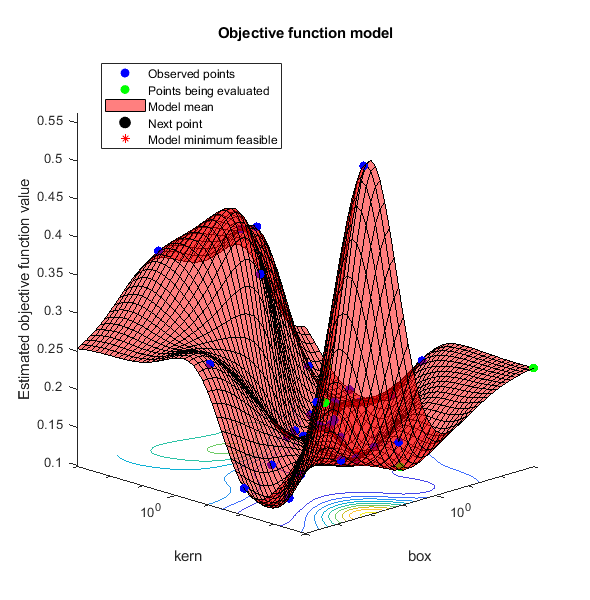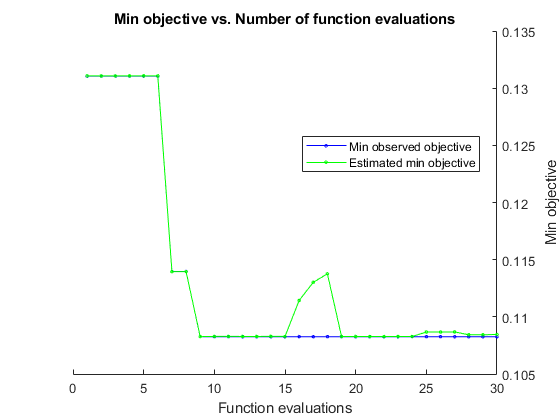```__________________________________________________________ Optimization completed. MaxObjectiveEvaluations of 30 reached. Total function evaluations: 30 Total elapsed time: 48.2085 seconds. Total objective function evaluation time: 128.3472 Best observed feasible point: box kern _________ _________ 0.0015925 0.0050225 Observed objective function value = 0.10826 Estimated objective function value = 0.10846 Function evaluation time = 4.0711 Best estimated feasible point (according to models): box kern _________ _________ 0.0015925 0.0050225 Estimated objective function value = 0.10846 Estimated function evaluation time = 2.8307 ```

Return the best feasible point in the Bayesian model `results` by using the `bestPoint` function. Use the default criterion `min-visited-upper-confidence-interval`, which determines the best feasible point as the visited point that minimizes an upper confidence interval on the objective function value.

`zbest = bestPoint(results)`
```zbest=1×2 table box kern _________ _________ 0.0015925 0.0050225 ```

The table `zbest` contains the optimal estimated values for the `'BoxConstraint'` and `'KernelScale'` name-value pair arguments. Use these values to train a new optimized classifier.

`Mdl = fitcsvm(X,Y,'BoxConstraint',zbest.box,'KernelScale',zbest.kern);`

Observe that the optimal parameters are in `Mdl`.

`Mdl.BoxConstraints(1)`
```ans = 0.0016 ```
`Mdl.KernelParameters.Scale`
```ans = 0.0050 ```

## Input Arguments

collapse all

Objective function, specified as a function handle or, when the `UseParallel` name-value pair is `true`, a `parallel.pool.Constant` (Parallel Computing Toolbox) whose `Value` is a function handle. Typically, `fun` returns a measure of loss (such as a misclassification error) for a machine learning model that has tunable hyperparameters to control its training. `fun` has these signatures:

```objective = fun(x) % or [objective,constraints] = fun(x) % or [objective,constraints,UserData] = fun(x)```

`fun` accepts `x`, a 1-by-`D` table of variable values, and returns `objective`, a real scalar representing the objective function value `fun(x)`.

Optionally, `fun` also returns:

• `constraints`, a real vector of coupled constraint violations. For a definition, see Coupled Constraints. `constraint(j) > 0` means constraint `j` is violated. ```constraint(j) < 0``` means constraint `j` is satisfied.

• `UserData`, an entity of any type (such as a scalar, matrix, structure, or object). For an example of a custom plot function that uses `UserData`, see Create a Custom Plot Function.

For details about using `parallel.pool.Constant` with `bayesopt`, see Placing the Objective Function on Workers.

Example: `@objfun`

Data Types: `function_handle`

Variable descriptions, specified as a vector of `optimizableVariable` objects defining the hyperparameters to be tuned.

Example: `[X1,X2]`, where `X1` and `X2` are `optimizableVariable` objects

### Name-Value Arguments

Specify optional pairs of arguments as `Name1=Value1,...,NameN=ValueN`, where `Name` is the argument name and `Value` is the corresponding value. Name-value arguments must appear after other arguments, but the order of the pairs does not matter.

Before R2021a, use commas to separate each name and value, and enclose `Name` in quotes.

Example: `results = bayesopt(fun,vars,'AcquisitionFunctionName','expected-improvement-plus')`

Algorithm Control

collapse all

Function to choose next evaluation point, specified as one of the listed choices.

Acquisition functions whose names include `per-second` do not yield reproducible results because the optimization depends on the runtime of the objective function. Acquisition functions whose names include `plus` modify their behavior when they are overexploiting an area. For more details, see Acquisition Function Types.

Example: `'AcquisitionFunctionName','expected-improvement-per-second'`

Specify deterministic objective function, specified as `false` or `true`. If `fun` is stochastic (that is, `fun(x)` can return different values for the same `x`), then set `IsObjectiveDeterministic` to `false`. In this case, `bayesopt` estimates a noise level during optimization.

Example: `'IsObjectiveDeterministic',true`

Data Types: `logical`

Propensity to explore, specified as a positive real. Applies to the `'expected-improvement-plus'` and `'expected-improvement-per-second-plus'` acquisition functions. See Plus.

Example: `'ExplorationRatio',0.2`

Data Types: `double`

Fit Gaussian Process model to `GPActiveSetSize` or fewer points, specified as a positive integer. When `bayesopt` has visited more than `GPActiveSetSize` points, subsequent iterations that use a GP model fit the model to `GPActiveSetSize` points. `bayesopt` chooses points uniformly at random without replacement among visited points. Using fewer points leads to faster GP model fitting, at the expense of possibly less accurate fitting.

Example: `'GPActiveSetSize',80`

Data Types: `double`

Compute in parallel, specified as `false` (do not compute in parallel) or `true` (compute in parallel). Computing in parallel requires Parallel Computing Toolbox™.

`bayesopt` performs parallel objective function evaluations concurrently on parallel workers. For algorithmic details, see Parallel Bayesian Optimization.

Example: `'UseParallel',true`

Data Types: `logical`

Imputation method for parallel worker objective function values, specified as `'clipped-model-prediction'`, `'model-prediction'`, `'max-observed'`, or `'min-observed'`. To generate a new point to evaluate, `bayesopt` fits a Gaussian process to all points, including the points being evaluated on workers. To fit the process, `bayesopt` imputes objective function values for the points that are currently on workers. `ParallelMethod` specifies the method used for imputation.

• `'clipped-model-prediction'` — Impute the maximum of these quantities:

• Mean Gaussian process prediction at the point `x`

• Minimum observed objective function among feasible points visited

• Minimum model prediction among all feasible points

• `'model-prediction'` — Impute the mean Gaussian process prediction at the point `x`.

• `'max-observed'` — Impute the maximum observed objective function value among feasible points.

• `'min-observed'` — Impute the minimum observed objective function value among feasible points.

Example: `'ParallelMethod','max-observed'`

Tolerance on the number of active parallel workers, specified as a positive integer. After `bayesopt` assigns a point to evaluate, and before it computes a new point to assign, it checks whether fewer than `MinWorkerUtilization` workers are active. If so, `bayesopt` assigns random points within bounds to all available workers. Otherwise, `bayesopt` calculates the best point for one worker. `bayesopt` creates random points much faster than fitted points, so this behavior leads to higher utilization of workers, at the cost of possibly poorer points. For details, see Parallel Bayesian Optimization.

Example: `'MinWorkerUtilization',3`

Data Types: `double`

Starting and Stopping

collapse all

Objective function evaluation limit, specified as a positive integer.

Example: `'MaxObjectiveEvaluations',60`

Data Types: `double`

Time limit, specified as a positive real. The time limit is in seconds, as measured by `tic` and `toc`.

Run time can exceed `MaxTime` because `bayesopt` does not interrupt function evaluations.

Example: `'MaxTime',3600`

Data Types: `double`

Number of initial evaluation points, specified as a positive integer. `bayesopt` chooses these points randomly within the variable bounds, according to the setting of the `Transform` setting for each variable (uniform for `'none'`, logarithmically spaced for `'log'`).

Example: `'NumSeedPoints',10`

Data Types: `double`

Constraints

collapse all

Deterministic constraints on variables, specified as a function handle.

For details, see Deterministic Constraints — XConstraintFcn.

Example: `'XConstraintFcn',@xconstraint`

Data Types: `function_handle`

Conditional variable constraints, specified as a function handle.

For details, see Conditional Constraints — ConditionalVariableFcn.

Example: `'ConditionalVariableFcn',@condfun`

Data Types: `function_handle`

Number of coupled constraints, specified as a positive integer. For details, see Coupled Constraints.

Note

`NumCoupledConstraints` is required when you have coupled constraints.

Example: `'NumCoupledConstraints',3`

Data Types: `double`

Indication of whether coupled constraints are deterministic, specified as a logical vector of length `NumCoupledConstraints`. For details, see Coupled Constraints.

Example: `'AreCoupledConstraintsDeterministic',[true,false,true]`

Data Types: `logical`

Reports, Plots, and Halting

collapse all

Command-line display level, specified as `0`, `1`, or `2`.

• `0` — No command-line display.

• `1` — At each iteration, display the iteration number, result report (see the next paragraph), objective function model, objective function evaluation time, best (lowest) observed objective function value, best (lowest) estimated objective function value, and the observed constraint values (if any). When optimizing in parallel, the display also includes a column showing the number of active workers, counted after assigning a job to the next worker.

The result report for each iteration is one of the following:

• `Accept` — The objective function returns a finite value, and all constraints are satisfied.

• `Best` — Constraints are satisfied, and the objective function returns the lowest value among feasible points.

• `Error` — The objective function returns a value that is not a finite real scalar.

• `Infeas` — At least one constraint is violated.

• `2` — Same as `1`, adding diagnostic information such as time to select the next point, model fitting time, indication that "plus" acquisition functions declare overexploiting, and parallel workers are being assigned to random points due to low parallel utilization.

Example: `'Verbose',2`

Data Types: `double`

Function called after each iteration, specified as a function handle or cell array of function handles. An output function can halt the solver, and can perform arbitrary calculations, including creating variables or plotting. Specify several output functions using a cell array of function handles.

There are two built-in output functions:

You can write your own output functions. For details, see Bayesian Optimization Output Functions.

Example: `'OutputFcn',{@saveToFile @myOutputFunction}`

Data Types: `cell` | `function_handle`

File name for the `@saveToFile` output function, specified as a character vector or string scalar. The file name can include a path, such as `'../optimizations/September2.mat'`.

Example: `'SaveFileName','September2.mat'`

Data Types: `char` | `string`

Variable name for the `@assignInBase` output function, specified as a character vector or string scalar.

Example: `'SaveVariableName','September2Results'`

Data Types: `char` | `string`

Plot function called after each iteration, specified as `'all'`, a function handle, or a cell array of function handles. A plot function can halt the solver, and can perform arbitrary calculations, including creating variables, in addition to plotting.

Specify no plot function as `[]`.

`'all'` calls all built-in plot functions. Specify several plot functions using a cell array of function handles.

The built-in plot functions appear in the following tables.

Model Plots — Apply When D ≤ 2Description
`@plotAcquisitionFunction`

Plot the acquisition function surface.

`@plotConstraintModels`

Plot each constraint model surface. Negative values indicate feasible points.

Also plot a P(feasible) surface.

Also plot the error model, if it exists, which ranges from `–1` to `1`. Negative values mean that the model probably does not error, positive values mean that it probably does error. The model is:

Plotted error = 2*Probability(error) – 1.

`@plotObjectiveEvaluationTimeModel`

Plot the objective function evaluation time model surface.

`@plotObjectiveModel`

Plot the `fun` model surface, the estimated location of the minimum, and the location of the next proposed point to evaluate. For one-dimensional problems, plot envelopes one credible interval above and below the mean function, and envelopes one noise standard deviation above and below the mean.

Trace Plots — Apply to All DDescription
`@plotObjective`

Plot each observed function value versus the number of function evaluations.

`@plotObjectiveEvaluationTime`

Plot each observed function evaluation run time versus the number of function evaluations.

`@plotMinObjective`

Plot the minimum observed and estimated function values versus the number of function evaluations.

`@plotElapsedTime`

Plot three curves: the total elapsed time of the optimization, the total function evaluation time, and the total modeling and point selection time, all versus the number of function evaluations.

You can write your own plot functions. For details, see Bayesian Optimization Plot Functions.

Note

When there are coupled constraints, iterative display and plot functions can give counterintuitive results such as:

• A minimum objective plot can increase.

• The optimization can declare a problem infeasible even when it showed an earlier feasible point.

The reason for this behavior is that the decision about whether a point is feasible can change as the optimization progresses. `bayesopt` determines feasibility with respect to its constraint model, and this model changes as `bayesopt` evaluates points. So a “minimum objective” plot can increase when the minimal point is later deemed infeasible, and the iterative display can show a feasible point that is later deemed infeasible.

Example: `'PlotFcn','all'`

Data Types: `char` | `string` | `cell` | `function_handle`

Initialization

collapse all

Initial evaluation points, specified as an `N`-by-`D` table, where `N` is the number of evaluation points, and `D` is the number of variables.

Note

If only `InitialX` is provided, it is interpreted as initial points to evaluate. The objective function is evaluated at `InitialX`.

If any other initialization parameters are also provided, `InitialX` is interpreted as prior function evaluation data. The objective function is not evaluated. Any missing values are set to `NaN`.

Data Types: `table`

Objective values corresponding to `InitialX`, specified as a length-`N` vector, where `N` is the number of evaluation points.

Example: `'InitialObjective',[17;-3;-12.5]`

Data Types: `double`

Constraint violations of coupled constraints, specified as an `N`-by-`K` matrix, where `N` is the number of evaluation points and `K` is the number of coupled constraints. For details, see Coupled Constraints.

Data Types: `double`

Errors for `InitialX`, specified as a length-`N` vector with entries `-1` or `1`, where `N` is the number of evaluation points. Specify `-1` for no error, and `1` for an error.

Example: `'InitialErrorValues',[-1,-1,-1,-1,1]`

Data Types: `double`

Initial data corresponding to `InitialX`, specified as a length-`N` cell vector, where `N` is the number of evaluation points.

Example: `'InitialUserData',{2,3,-1}`

Data Types: `cell`

Evaluation times of objective function at `InitialX`, specified as a length-`N` vector, where `N` is the number of evaluation points. Time is measured in seconds.

Data Types: `double`

Times for the first `N` iterations, specified as a length-`N` vector, where `N` is the number of evaluation points. Time is measured in seconds.

Data Types: `double`

## Output Arguments

collapse all

Bayesian optimization results, returned as a `BayesianOptimization` object.

collapse all

### Coupled Constraints

Coupled constraints are those constraints whose value comes from the objective function calculation. See Coupled Constraints.

## Tips

• Bayesian optimization is not reproducible if one of these conditions exists:

• You specify an acquisition function whose name includes `per-second`, such as `'expected-improvement-per-second'`. The `per-second` modifier indicates that optimization depends on the run time of the objective function. For more details, see Acquisition Function Types.

• You specify to run Bayesian optimization in parallel. Due to the nonreproducibility of parallel timing, parallel Bayesian optimization does not necessarily yield reproducible results. For more details, see Parallel Bayesian Optimization.

## Version History

Introduced in R2016b